Elias Pampalk - MA Toolbox - Documentation

Overview

The Matlab functions and scripts in the MA toolbox are:

 - ma_g1c_ExtractFeatures extract the features necessary to compute the G1C distance (using the MIREX'06 input format) - ma_g1c_ComputeSimilarities compute the G1C distance (using MIREX'06 output format) - ma_sone wav (PCM) to sone (specific loudness sensation) - ma_mfcc wav (PCM) to MFCCs (Mel Frequency Cepstrum Coefficients) - ma_sh sone to Spectrum Histogram - ma_ph sone to Periodicity Histogram - ma_fp sone to Fluctuation Pattern - ma_fc frame based representation (MFCCs or sone) to cluster model (Frame Clustering) - ma_cms cluster models to distance (Cluster Model Similarity) - ma_kmeans kmeans clustering (used by "ma_fc") - ma_cm_visu visualize a cluster model (as returned by "ma_fc") - ma_simple_eval script for a simple evaluation of similarity measures - ma_simple_iom script for a simple islands of music interface

Most of the functions run a simple test when called without an input argument. These built-in tests are an easy way to play around with the parameters.

Most functions have an input argument called "p". This is a Matlab structure variable and is used to store all parameter values conveniently. See the test methods on how to set them, in some cases default values are defined.

To use the functions it is necessary to load the WAV (PCM) files using Matlab's "wavread ".

ma_g1c_FeatureExtraction

%%         G1C, implementation as described in thesis
%%         for mirex'06, "music audio search" (was audio music similarity)
%%
%% USAGE EXAMPLES
%%       FeatureExtraction(in_file,out_dir)
%%       FeatureExtraction('mypath/myInputFile.txt','mypath/myOutputDirectory')
%%       FeatureExtraction('mypath/myInputFile','mypath/myOutputDirectory/')
%%
%% INPUT ARGUMENTS
%%
%% in_file: path fo file containing list of wav files to extract features from
%%          all files are 22050Hz mono wav (this function checks if this is true)
%% out_dir: directory to which this function can write output. (logfiles,
%%          and extracted features), files written to out_dir must be read
%%          by function "DistanceCumputation"
%%
%% in_file format (text):
%%      path/to/audiofile/0000001.wav
%%      path/to/audiofile/0000002.wav
%%      path/to/audiofile/0000003.wav
%%      ...
%%      path/to/audiofile/9999999.wav

ma_g1c_ComputeSimilarities

%%         G1C, implementation as described in thesis
%%         for mirex, "music audio search" (was audio music similarity)
%%
%% USAGE EXAMPLE
%%       FeatureExtraction(in_file,out_dir)
%%       ComputeSimilarities(out_dir,'somepath/distance_matrix.txt')
%%
%% INPUT ARGUMENTS
%%
%% in_dir:   directory to which function "FeatureExtraction" was writing to.
%%           a log file will be created in this directory
%% out_file: whole distance matrix in the following format
%%
%%
%%
%%     1   path\file1.wav
%%     2   path\file2.wav
%%     ...
%%     N  path\fileN.wav
%%     Q\R   1       2      ...      N
%%     1     0.000  10.234  ...   123.32
%%     2    10.234   0.000  ...    23.45
%%     .     ...     ...    0.000   ...
%%     N     4.1234  6.345  ...     0.0
%%
%%
%%   delimiter: tabulator space, number format: float

ma_sone

Estimates the loudness sensation per frequency band using auditory models. The main components are (1) outer-ear model, (2) critical-band rate scale (Bark-scale), (3) spectral masking, and (4) Sone.
Compute sonogram for a pcm signal.
[sone, Ntot, p] = ma_sone(wav,p)

USAGE
ma_sone;                %% uses test-sound and creates figures
sone = ma_sone(wav,p);  %% normal usage
[sone, Ntot, p] = ma_sone(wav,p);

INPUT
wav (vector) obtained from wavread (use mono input! 11kHz recommended)
p (struct) parameters e.g.
p.fs          = 11025;     %% sampling frequency of given wav (unit: Hz)
* p.do_visu     = 0;         %% create some figures
* p.do_sone     = 1;         %% compute sone (otherwise dB)
* p.outerear = 'terhardt';   %% outer ear model {'terhardt' | 'none'}
* p.fft_size = 256;          %% window size (unit: samples)
%% 256 are ~23ms @ 11kHz
* p.hopsize  = 128;          %% fft window hopsize (unit: samples)
* p.bark_type   = 'table';   %% type of bark scale to use (either:
%% 'table' lookup (=default) with max 24 bands
%% (for 44kHz), or
%% vector
[min_freq max_freq num_bands]
* p.dB_max      = 96;        %% max dB of input wav
%% (for 16 bit input 96dB is SPL)

all fields marked with * are optional (defaults are defined)

OUTPUT
sone (matrix) rows are bark bands, columns are time intervalls,
values are sone (or dB)
Ntot (vector) total loudness (stevens method, see "signal sound
and sensation" p73, hartmann)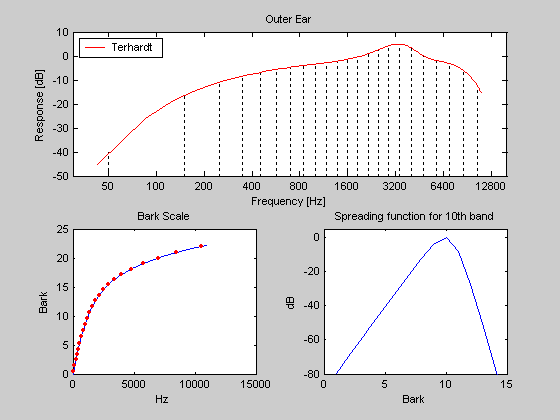Figure 1. The main characteristics of the sone model. The upper subplot shows the width of the critical-bands and the outer-ear model. The lower left shows the relationship between the Bark-scale and Hz. The lower right shows the spectral masking function. Some things to notice: The Bark-scale is linear up to about 500Hz. The spectral masking is not symmetric. Higher tones are masked stronger by lower ones than vice versa. The outer-ear is most responsive to frequencies around 3-4kHz. For details on the models see the source code (e.g. "type ma_sone").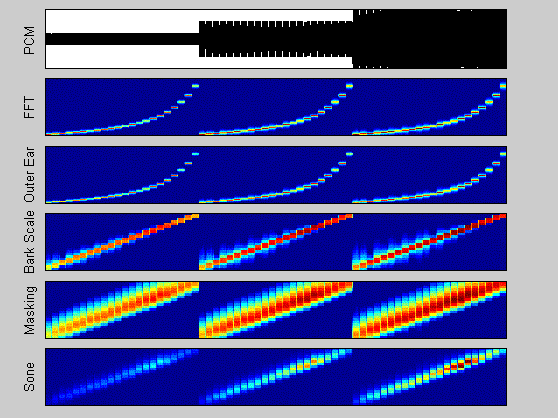Figure 2. The characteristics of the sone model can be demonstrated with a simple sine-tone. The tone is generated at different frequencies (center frequencies of the critical-bands) with fixed amplitude (3 times, each time the fixed amplitude is increased). Note the difference between the linear frequency scale (used in the outer-ear and FFT representations) and the Bark-scale (the lower 3 subplots). The sinusoids in each sweep from low to high have the same amplitudes, they are not perceived equally loud (uncomment the "sound(wav,p.fs)" line in the test method code to hear the sound, this pitch dependent loudness sensation is reflected in the model (in contrast to the MFCC model).

ma_mfcc

Mel Frequency Cepstrum Coefficients are very popular in speech processing. They are based on the Mel-scale. The Mel scale is defined such that a tone of 1000Hz equals 1000mel. If tone A is perceived twice as high as tone B then its mel value is twice as high. (The Bark-scale and in particular the width of the critical-bands are defined by masking tresholds, i.e., how close can two tones be too each other on the frequency scale so that they are both perceived and one doesn't mask the other).
In addition to using the Mel-scale, dB values are computed and the spectrum is compressed (and smoothed) using a Discrete Cosine Transform (DCT). This compressed representation is well suited for computational heavy algorithms. (Note that a DCT compression could also be applied to the sonogram.)

Compute Mel Frequency Cepstrum Coefficients.
[mfcc,DCT] = ma_mfcc(wav, p)

USAGE
ma_mfcc;                         %% test mode
mfcc = ma_mfcc(wav, p);          %% normal usage
[mfcc, DCT] = ma_mfcc(wav, p);   %% DCT matrix is needed to decode the
%% coefficients (e.g. for visualization)

based on function "mfcc" in Auditory Toolbox by Malcolm Slaney
(http://www.slaney.org/malcom) see Auditory Toolbox for details

INPUT
(use mono input! 11kHz recommended)
p (struct) parameters e.g.
p.fs              = 11025; %% sampling frequency of given wav (unit: Hz)
* p.visu            = 0;     %% create some figures
* p.fft_size        = 256;   %% (unit: samples) 256 are about 23ms @ 11kHz
* p.hopsize         = 128;   %% (unit: samples) aka overlap
* p.num_ceps_coeffs = 20;
* p.use_first_coeff = 1;     %% aka 0th coefficient (contains information
%% on average loudness)

* p.mel_filt_bank   = 'auditory-toolbox';
%% mel filter bank choice
%% {'auditory-toolbox' | [f_min f_max num_bands]}
%% e.g. [20 16000 40], (default)
%% note: auditory-toobox is optimized for
%%       speech (133Hz...6.9kHz)

* p.dB_max      = 96;        %% max dB of input wav
%% (for 16 bit input 96dB is SPL)
all fields marked with * are optional (defaults are defined)

OUTPUT
mfcc (matrix) size coefficients x frames
DCT (matrix)Figure 3. The basic model of the MFCCs. The upper subplot shows the filterbank of triangular filters. The lower subplot shows the Discrete Cosine Transform matrix.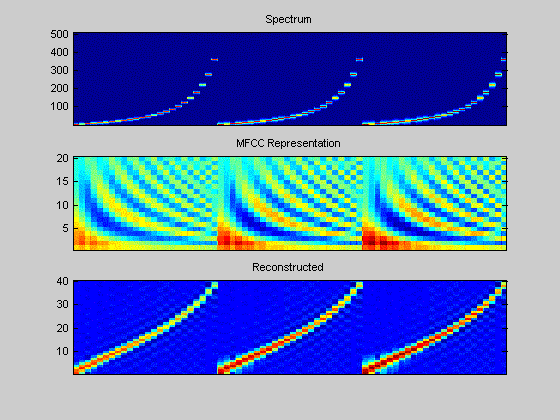Figure 4. Using the same input sound as in Figure 2 the characteristics of the MFCC can be studied. Some things to notice: The mel-scale differs from the bark-scale (the line is not straight). Lower frequencies have higher values. Artefacts from the DCT transform are clearly visible.

ma_sh

The Spectrum Histogram is a very simple approach to summarize the variations in the dynamics. It counts for each frequency band how many times certain loudness levels are exceeded.

compute Spectrum Histogram (SH)
very simple approach to summarize the sonogram
see Pampalk et al. ISMIR'03 for details

sh = ma_sh(sone,hist_res)

USAGE
sone = ma_sone(wav,p);
sh = ma_sh(sone,hist_res)

INPUT
sone (matrix) as returned from "ma_sone" (size: critical-bands x time)
hist_res = 25; %% loudness resolution in histogram (used 50 for ismir03)

OUTPUT
sh (matrix) size: critical-bands x hist_res

note: to compute distances between two SHs use Euclidean distance, e.g.
distance = norm(sh1(:)-sh2(:));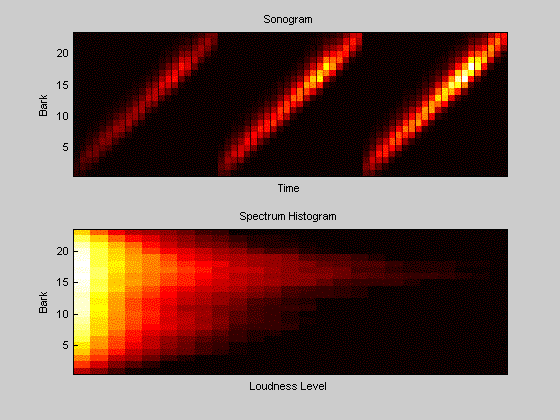Figure 5. Same test sound as in the examples above. The spectrum histogram counts how often a loudness level was exceeded in each critical-band.

ma_ph

The Periodicity Histogram is based on a comb-filter and describes periodically reoccurring sharp attacks in the audio. Although the PH is not a useful similarity measure it serves as a good starting point to compute various descriptors.

compute Periodicity Histogram (PH)
see Pampalk et al. ISMIR'03 for details

[ph, ph_low, p] = ma_ph(sone,p)

INPUT
sone (matrix) as returned from "ma_sone" (size: critical-bands x time)
parameter structure p
p.hist_res = 30; energy resolution in histogram (used 50 for ismir03)
p.sequence.length = 1024; %% ~12 sec @ 11025, size of sequence (window) to
%% analyze periodicities
p.sequence.hopsize = 512; %% smaller values make sense,
p.sequence.windowfunction = 'hann';
p.fft_hopsize = 256; %% (~12ms @ 11kHz) hopsize used to create
%% sonogram (see ma_sone)
p.acc = 5;       %% ismir version orig: 5 (recommended for 12ms hopsize in
%% sone), combfilter accuracy
p.minbpm = 40;   %% ismir version orig: 40, minimum bpm to look for
p.maxbpm = 240;  %% ismir version orig: 240
p.hist_res = 30; %% resolution of histogram
p.maxval = 0.4;  %% highest energy to expect in histogram
p.minval = 0;    %% 1/p.hist_res
p.visu = 0;      %% do some visualizations
p.preferred_tempo = 'moleants'; %% {'moleants' | 'none'}

OUTPUT
ph (matrix) size: critical-bands x hist_res
ph_low (matrix), same as ph but only for the lowest frequency bands

note: to compute distances between two PHs use Euclidean distance, e.g.
distance = norm(ph1(:)-ph2(:));
however, PHs best serve as starting point for higher level descriptors e.g.
using sum(ph(:)) (= total energy contained in periodic beats)Figure 6. The different steps in computing the periodicities for a 12sec time frame. The input is a very simple test sound generated with "ma_test_create_wav".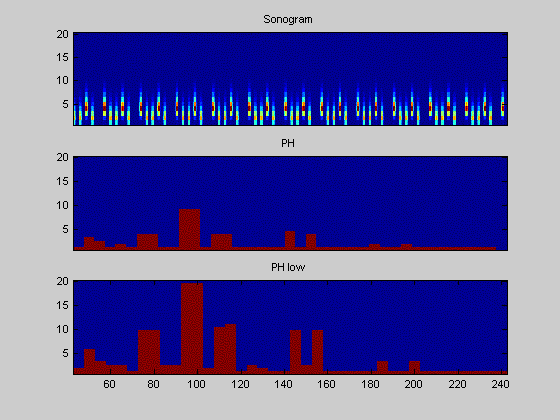Figure 7. If the input is long enough, then the results from each 12sec frame are summarized in a histogram, counting for each periodicity (bpm) how often specific energy levels were exceeded.

ma_fp

The Fluctuation Pattern is based on a model of the perceived fluctuation for amplitude modulated tones.

compute Fluctuation Pattern (FP)
see Pampalk et al. ACM-MM'02 for details
december 2001 version (islands of music, master's thesis)

fp = ma_fp(sone,p)

INPUT
sone (matrix) as returned from "ma_sone" (size: critical-bands x time)
parameter structure p
p.sequence.length = 512;   %% windows size (ca 6sec @ 11kHz with
%% 128 sone hopsize)

p.sequence.hopsize = 256;
p.sequence.windowfunction = 'boxcar';
p.fs = 11025;              %% sampling frequency of wav file
p.fft_hopsize = 128;       %% (~12ms @ 11kHz) hopsize used to create sone
p.visu = 0;                %% do some visualizations
OUTPUT
fp (matrix) size: critical-bands x 60 (modulation frequencies)

note: to compute distances between two FPs use Euclidean distance, e.g.
distance = norm(fp1(:)-fp2(:));Figure 8. The different steps in computing the fluctuation pattern for a 6sec sequence.

ma_fc

Frame Clustering takes a frame based representation as input (e.g. MFCCs) and creates a cluster model. The cluster models can be compared to each other with "ma_cms" (Cluster Model Similarity).
A variation using the Earth Mover's Distance was originally published by Beth Logan and Ariel Salomon (2001). A second variation using monte carlo sampling was publised by Jean-Julien Aucouturier and Francois Pachet (2002).

To run "ma_fc" with the "gmm" (Gaussian Mixture Model) option, the directory of the Netlab toolbox must be added to the Matlab path (e.g. "addpath ../netlab").

compute Frame-based Clustering (FC)
see Beth Logan and Ariel Salomon, ICME'01
and Jean-Julien Aucouturier and Francois Pachet, ISMIR'02
for details

frames = ma_mfcc(wav,p); or frames = ma_sone(wav,p);
cm = ma_fc(frames,p);
distance = ma_cms(cm1,cm2,p);

specific parameters for k-means and gmm need to be set in the code.

INPUT
frames (matrix) as returned from "ma_sone" or "ma_mfcc"
(size: frequency-bands x time)
parameter structure p
p.cluster_type = 'kmeans'; %% {'kmeans' | 'gmm'}
%% 'gmm' requires NETLAB toolbox
p.num_clusters = 3;        %% number of clusters
p.covar_type   = 'diag';   %% {'spherical' | 'diag' | 'full'}

OUTPUT
cm (structure) cluster model (netlab gmm model structure)
type: 'gmm'
nin: 19
ncentres: 25
covar_type: 'full'
priors: [1x25 double]
centres: [25x19 double]
covars: [19x19x25 double]
nwts: 9525

note: to compute distances between two CMs use "ma_cms"
to visualize a CM use "ma_cm_visu"

For figures see "ma_cms".

ma_cms

Cluster Model Similarity takes the models computed by "ma_fc" as input and outputs a distance. There are basically two ways to compute the similarity: using the EMD-KL (Earth Movers Distance and Kullback Leibler Divergence), or by Monte Carlo sampling.

EMD-KL requires functions implemented by Yossi Rubner. Monte Carlo sampling requires the NETLAB toolbox.

compute Cluster Model Similarity (CMS)
see Beth Logan and Ariel Salomon, ICME 2001
(using Kulback Leibler Divergence and Earth Mover's Distance)
and Jean-Julien Aucouturier and Francois Pachet, ISMIR 2002 +
IEEE Workshop on Model Based Processing and Coding of Audio 2002
(using Gaussian Mixture Models and monte carlo sampling)
for details

USAGE
frames = ma_mfcc(wav,p);  or frames = ma_sone(wav,p);
cm = ma_fc(frames,p);
distance = ma_cms(cm1,cm2,p);

note: 'KL_EMD' needs functions supplied by Yossi Rubner
'monte_carlo' sampling needs netlab toolbox

if called without arguments, 3 test sounds are created using
"ma_test_create_wav" and the distances between these are computed

INPUT
cluster models (structure) as returned from "ma_fc"
parameter structure p
p.cm_similarity = 'monte_carlo';  %% {'KL_EMD' | 'monte_carlo'}
p.mc_samples = 2000;  %% only needed for 'monte_carlo'
OUTPUT
d (scalar) distance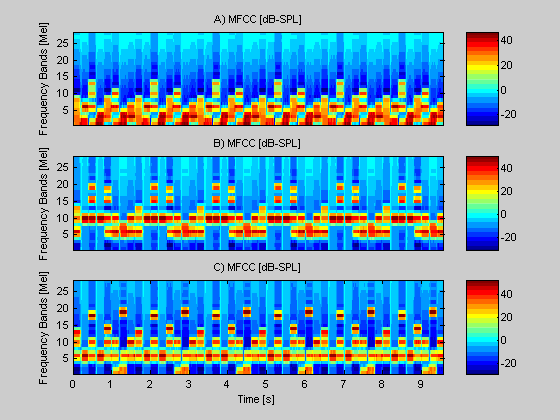Figure 9. The three sounds used in this test.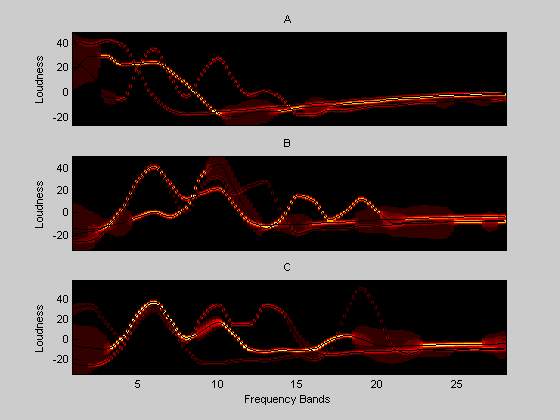Figure 10. The cluster models can be visualized using "ma_cm_visu". In this case each sound was represented by 3 Gaussian clusters. The shapes and variances of each clusters (cummulated probability) are shown.Figure 11. The distance matrix of the 3 sounds.

ma_kmeans

simple batch k-means

[C,Qe,N,W,Q] = ma_kmeans(X, iter, c, C)

INPUT
X    .. Data
iter .. Iterations (if set to 0, only initialize)
c    .. number of clusters
C    .. initial Codebooks (default = randperm select k)

OUTPUT
C    .. centers
Qe   .. final quantisation error
N    .. number of items mapped to each cluster
W    .. winner (best matching cluster for each item)
Q    .. quantisation error of each item (Qe = mean(Q))

used by ma_fc (frame clustering)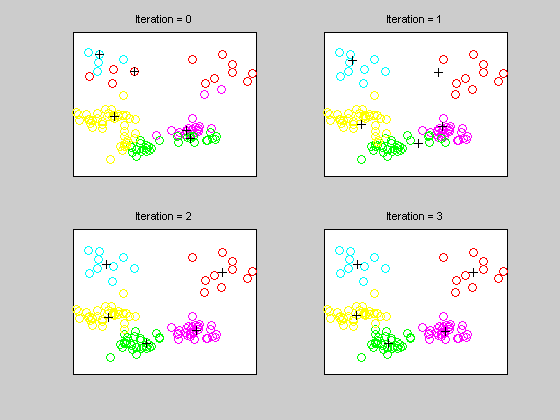ma_cm_visu

my_pdf = ma_cm_visu(cm,p,s)

visualize Cluster Model (CM)
see "ma_fc" for details

INPUT
cm (structure) as returned from "ma_fc"
parameter structure p
p.cluster_type = 'kmeans';  %% {'kmeans' | 'gmm'}
p.DCT (matrix) as returned from ma_mfcc,
if not given assume no DCT compression used
s (handle to subplot) optional, if given no new figure is created

some visualization parameters can only be set in the code

OUTPUT
my_pdf (matrix) dimensions: dB vs frequency band (same as visualized)

note: to compute distances between two CMs use "ma_cms"

See "ma_cms" for figures.

ma_simple

This is a simple script to demonstrate how to combine the different functions if the goal is to evaluate different parameter settings. Input is a bunch of wav files sorted into directories such that similar pieces are in the same directory. At the end the R-precision is computed.

ma_simple_iom

This is a simple script to demonstrate how the SOM toolbox (GNU-GPL) and the SDH toolbox (GNU-GPL) can be used to create "islands of music". (Both toolboxes need to be in the Matlab path.)